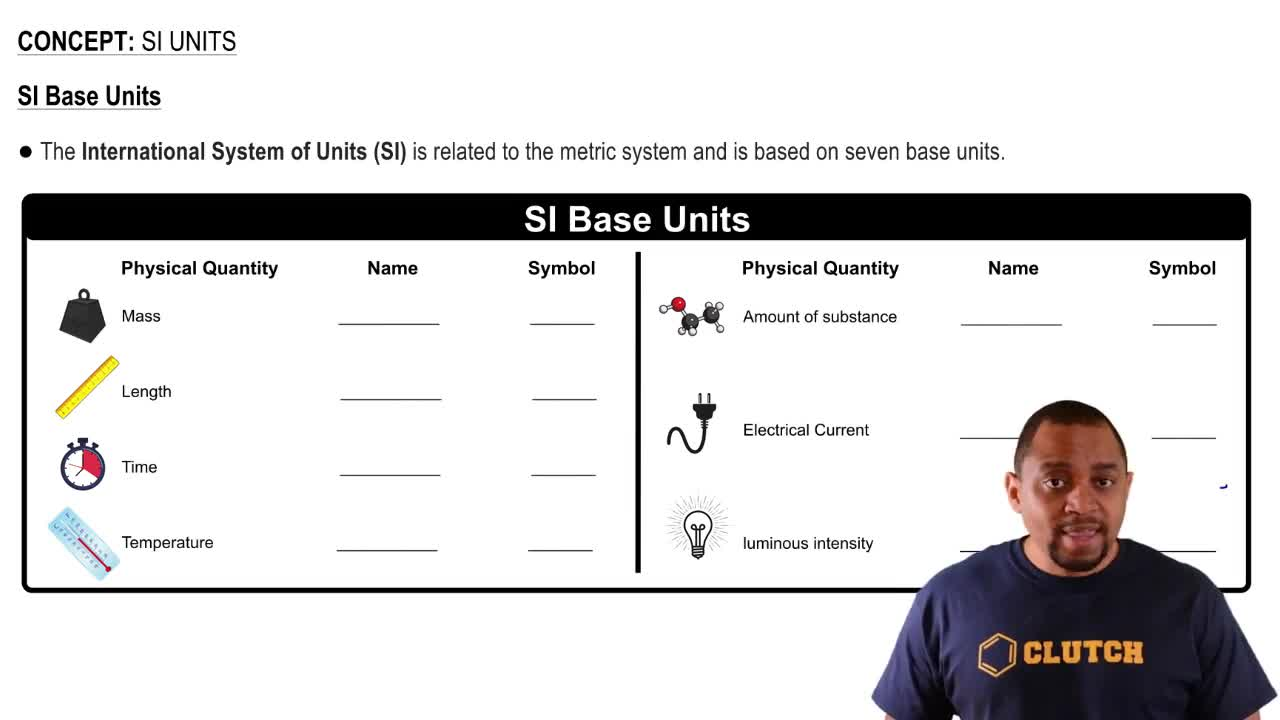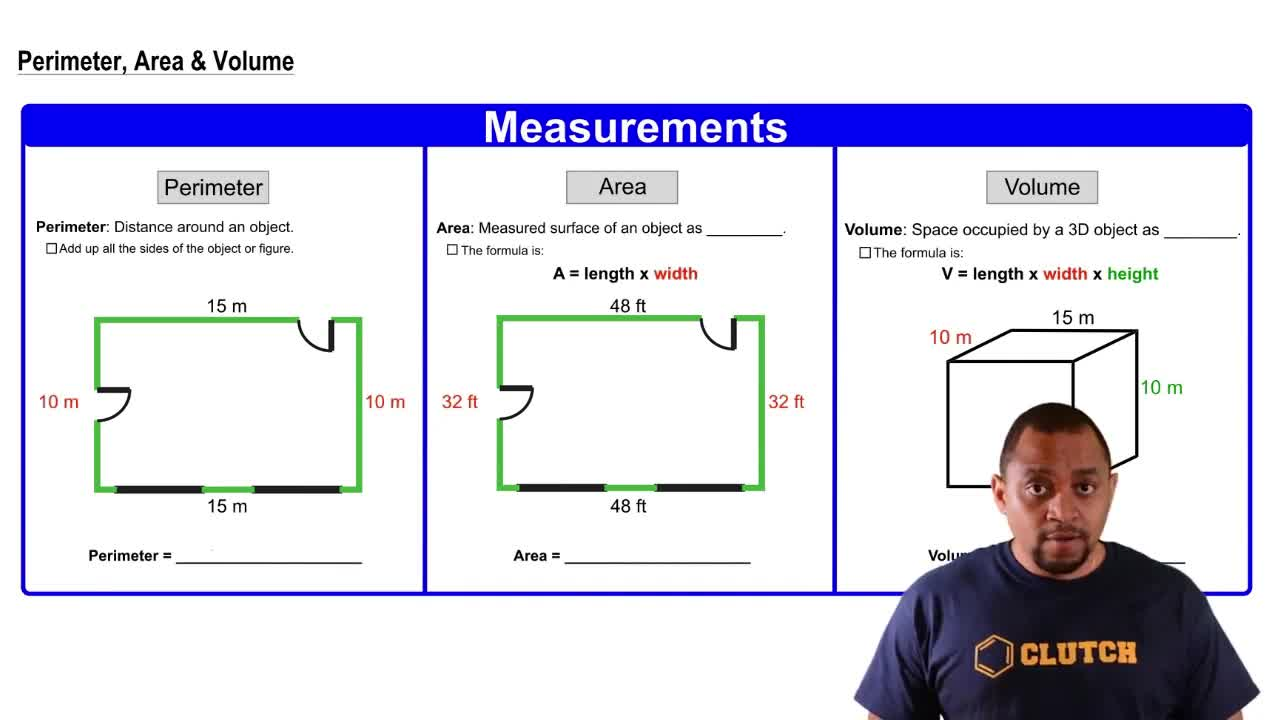Start typing, then use the up and down arrows to select an option from the list.
4:44 minutes
Problem 111
Textbook Question

# Force is defined as mass times acceleration. Starting with SI base units, derive a unit for force. Using SI prefixes, suggest a convenient unit for the force resulting from a collision with a 10-ton trailer truck moving at 55 mi per hour and for the force resulting from the collision of a molecule of mass around 10 - 20 kgmoving almost at the speed of light (3 * 108 m>s) with the wall of its container. (Assume a 1-second deceleration time for both collisions.)Verified Solution
This video solution was recommended by our tutors as helpful for the problem above.
95views